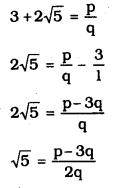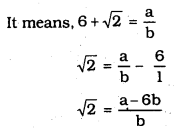# KSEEB SSLC Class 10 Maths Solutions Chapter 8 Real Numbers Ex 8.3

In this chapter, we provide KSEEB SSLC Class 10 Maths Solutions Solutions Chapter 8 Real Numbers Ex 8.3 for English medium students, Which will very helpful for every student in their exams. Students can download the latest KSEEB SSLC Class 10 Maths Solutions Solutions Chapter 8 Real Numbers Ex 8.3 pdf, free KSEEB SSLC Class 10 Maths Solutions Solutions Chapter 8 Real Numbers Ex 8.3 pdf download. Now you will get step by step solution to each question.

### Karnataka State Syllabus Class 10 Maths SolutionsSolutions Chapter 8 Real Numbers Ex 8.3

Question 1.
Prove that 5–√ is irrational.
Solution:
Let us assume, to the contrary, that 5–√ is rational.
∴ 5–√=ab
∴ b × 5–√ = a
By Squaring on both sides,
5b2 = a2 …………. (i)
∴ 5 divides a2.
5 divides a.
∴ We can write a = 5c.
Substituting the value of ‘a’ in eqn. (i),
5b2 = (5c)2 = 25c2
b2 = 5c2
It means 5 divides b2.
∴ 5 divides b.
∴ ‘a’ and ‘b’ have at least 5 as a common factor.
But this contradicts the fact that a’ and ‘b’ are prime numbers.
∴ 5–√ is an irrational number.

Question 2.
Prove that 3+25–√ is irrational.
Solution:
Let us assume that 3+25–√ is an irrational number.
Here, p, q, ∈ z, q ≠ 05–√ is rational number.
∵ p−3q2q is rational number.
But 5–√ is not a rational number.
∴ 3+25–√ is an irrational number.

Question 3.
Prove that the following are irrationals :
i) 12√
ii) 75–√
iii) 6+2–√
Solution:
i) Let 12√ is a rational number.
12√=pq
2–√=qp
By Squaring on both sides,
2 × p2 = q2
2, divides q2.
∴ 2, divides q
∵ q is an even number.
Similarly ‘p’ is an even number.
∴ p and q are even numbers.
∴ Common factor of p and q is 2.
This contradicts the fact that p and q also irrational.
∴ 2–√ is an irrational number.
∴ 12√ is an irrational number.

ii) Let 75–√is a rational number.
∴ 75–√=pq
5–√=p7q
Here,p7q is one rational number.
It means 5–√ which is equal also a rational number.
This contradicts to the fact that 5–√ is an irrational number.
This contradicts to the fact that 75–√ is rational number.
∴ 75–√ is a rational number.

iii) Let 6+2–√ is a rational number.a−6bb is a rational number, b
∴ 2–√ is also rational number.
This contradicts to the fact that 2–√ is an irrational number.
This contradicts to the fact that 6+2–√ is a rational number.
∴ 6+2–√ is an irrational number.

All Chapter KSEEB Solutions For Class 10 Maths

—————————————————————————–

All Subject KSEEB Solutions For Class 10

*************************************************

I think you got complete solutions for this chapter. If You have any queries regarding this chapter, please comment on the below section our subject teacher will answer you. We tried our best to give complete solutions so you got good marks in your exam.

If these solutions have helped you, you can also share kseebsolutionsfor.com to your friends.

Best of Luck!!